# MCQs on Electrical Machines

##### Page 113 of 114. Go to page 1 2 3 4 5 6 7 8 9 10 11 12 13 14 15 16 17 18 19 20 21 22 23 24 25 26 27 28 29 30 31 32 33 34 35 36 37 38 39 40 41 42 43 44 45 46 47 48 49 50 51 52 53 54 55 56 57 58 59 60 61 62 63 64 65 66 67 68 69 70 71 72 73 74 75 76 77 78 79 80 81 82 83 84 85 86 87 88 89 90 91 92 93 94 95 96 97 98 99 100 101 102 103 104 105 106 107 108 109 110 111 112 113 114
01․ For a DC machine having P no of pole pairs Z no of conductors having length L of resistivity ρ and cross section S are arranged. what is the armature resistance for lap-winding ?
PZL/4S
PZL/ 4Sa
PZL/4SP²
PZL/4Sa²

Resistance of each conductor = ρL/S and Z/2a conductors in series Resistance of each armature path = PLZ/2aS There are 2a Such resistances are in parallel in the armature ∴ Armature resistance = PZL/ 2aS×1/2a⇒ PZL/ 4Sa² For Simple lap winding 2a = 2P and a=P ∴ Armature resistance = PZL/4SP²

02․ For a DC machine having P no of pole pairs, Z no of conductors having length L of resistivity ρ and cross section S are arranged. what is the armature resistance for simple wave-winding ?
PZL/4S
PZL/ 4Sa
PZL/4Sa²
PZL/4Sp²

Resistance of each conductor = ρL/S and Z/2a conductors in series Resistance of each armature path = PLZ/2aS There are 2a Such resistances are in parallel in the armature ∴ Armature resistance = PZL/ 2aS×1/2a⇒ PZL/ 4Sa² For simple wave winding ⇒ 2a = 2, a=1. ∴ Armature resistance = PZL / 4S.

03․ A 200 V DC machine supplies 20 A at 200 V as a generator. The armature resistance is 0.2 Ω. If the machine is now operated as a motor at same terminal voltage and current but with the flux increased by 10%, the ratio of motor speed to generator speed is
0.87
0.95
0.96
1.06

200 V, 20 A and Ra=0.2 Ω. Eg = Vt+Ia×Ra = 200+20×0.2 = 204 V Eb = Vt-Ia×Ra = 200 - 20×0.2 = 196 V From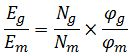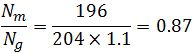04․ A 220 ,V 15 , kW 100 rpm shunt motor with armature resistance of 0.25 , W has a rated line current of 68 A and a rated field current of 2.2 A. The change in field flux required to obtain a speed of 1600 rpm while drawing a line current of 52.8 A and a field current of 1.8 A is
18.18% increase
36.36% increase
36.36% increase
36.36% decrease

From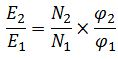φ21 = 0.6364. % decrease = [(φ12)/φ1] × 100 ⇒ 36.36% decrease.

05․ A 220 V, DC shunt motor is operating at a speed of 1440 rpm. The armature resistance is 1.0 Ω and armature current is 10 A. of the excitation of the machine is reduced by 10%, the extra resistance to be put in the armature circuit to maintain the same speed and torque will be
1.79 Ω
2 .1 Ω
1.8 9 Ω
3.1 Ω

Eb1 = 220 - 10×1⇒ 210 V. φ2 = 0.9×φ1 and Ia2 = 10/0.9 ⇒ 11.1 A FromEb2 = 189 V. Eb2 = Vt - Ia2×(Ra+Rse) Rse = 1.79 Ω.

06․ A separately excited DC motor runs at 1500 rpm under no-load with 200 V applied to the armature. The field voltage is maintained at its rated value. The speed of the motor, when it delivers a torque of 5 N-m, is 1400 rpm as shown in figure. The rotational losses and armature reaction are neglected. For the motor to deliver a torque of 2.5 N-m at 1400 rpm, the armature voltage to be applied is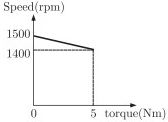125.5 V
193.3 V
200 V
241.7 V

No = 1500 rpm, Vt = 200 V, Te = 5 N-m, N1 = 1400 rpm. From, Eb2/ Eb1 = N2/ N1 Eb2 = 186.67 V. Te = Eb×Ia / ω, Ia = 3.926 A Ra= (Vt-Eb)/ Ia⇒ 3.4 Ω. for Te = 2.5 N-m, the voltage applied is 193.3 V.

07․ A 240 V, dc shunt motor draws 15 A while supplying the rated load at a speed of 80 rad/s. The armature resistance is 0.5 W and the field winding resistance is 80 W. The net voltage across the armature resistance at the time of plugging will be
6 V
234 V
240 V
474 V

Given Vt = 240 V, I = 15 A, Ra = 0.5 Ω, Rsh = 80 Ω. Ish = 240/80 ⇒ 3 A. Ia = 15-3 ⇒ 12 A. Eb = 240-12×0.5 ⇒ 234 V. Vplugging = Vt+ Eb = 240+234 ⇒ 474 V.

08․ A 8-pole, DC generator has a simplex wave-wound armature containing 32 coils of 6 turns each. Its flux per pole is 0.06 Wb. The machine is running at 250 rpm. The induced armature voltage is
96 V
192 V
384 V
768 V

N = 250 rpm, Z = 2×32×6=384, A = 2, P=8, φ = 0.06 Wb From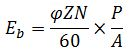Eg = 384 V.

09․ A dc series motor driving and electric train faces a constant power load. It is running at rated speed and rated voltage. If the speed has to be brought down to 0.25 p.u. the supply voltage has to be approximately brought down to
0.75 p.u
0.5 p.u
0.25 p.u
0.125 p.u

A Series Motor driving a constant power load running at rated speed and rated voltage. Eb = Vt - Ia×(Ra+Rse) Eb = K×φ×N, N = Eb / K×φ. As in series motor φ ∝ Ia, N = Eb / K×Ia. Te ∝ φ×Ia ∝ (Ia)². At constant power load, Eb×Ia = Te×ω = constant. If ω is decreased to 1/4 then Torque is increased to 4 times. So the voltage is brought down to 0.5 p.u.

10․ A 240 V, dc shunt motor draws 15 A while supplying the rated load at a speed of 80 rad/s. The armature resistance is 0.5 W and the field winding resistance is 80 W. The external resistance to be added in the armature circuit to limit the armature current to 125% of its rated value is
31.1 Ω
31.9 Ω
15.1 Ω
15.9 Ω

External resistance to be added in the armature circuit to limit the armature current upto 125%.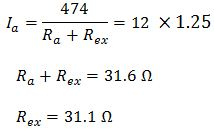<<<111112113114>>>